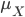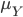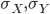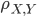×
Get Full Access to Statistics For Engineers And Scientists - 4 Edition - Chapter 2.6 - Problem 4e
Get Full Access to Statistics For Engineers And Scientists - 4 Edition - Chapter 2.6 - Problem 4e

×

# In a piston assembly, the specifications for the clearanceISBN: 9780073401331 38

## Solution for problem 4E Chapter 2.6

Statistics for Engineers and Scientists | 4th Edition

• Textbook Solutions
• 2901 Step-by-step solutions solved by professors and subject experts
• Get 24/7 help from StudySoup virtual teaching assistantsStatistics for Engineers and Scientists | 4th Edition

4 5 1 409 Reviews
12
5
Problem 4E

In a piston assembly, the specifications for the clearance between piston rings and the cylinder wall are very tight. In a lot of assemblies, let X be the number with too little clearance and let Y be the number with too much clearance. The joint probability mass function of X and Y is given in the table below:

Step-by-Step Solution:

Step 1 of 2

Given, the number with too little clearance is X and

The number with too much clearance is Y.

Then the joint probability mass function of X and Y is given in the table below.

 y x 0 1 2 3 0 0.15 0.12 0.11 0.1 1 0.09 0.07 0.05 0.04 2 0.06 0.05 0.04 0.02 3 0.04 0.03 0.02 0.01

Our goal is:

a). Find the probability mass function of X.

b). Find the probability mass function of Y.

c). Are X and Y are not independent? Explain.

d). Find meanand.

e). Find.

f). Find Cov (X,Y).

g). Find.

Step 2 of 2

## Discover and learn what students are asking

Statistics: Informed Decisions Using Data : Applications of the Normal Distribution
?Explain why P(X ? 220) should be reported as > 70.9999 if X is a normal random variable with mean 100 and standard deviation 15.

Unlock Textbook Solution